# Creating Custom Functions

Once you assign a value to a variable, you can use that value in any expression. For example, after you assign the number 25 to the variable myAge, the value of the conditional expression myAge > 20 would be True because 25 is greater than 20. You use the variable as if it were the value that it contains.

A function is like a variable, except that a function is smarter. A function is a variable that figures out its own value whenever you use it. For example, Microsoft Visual Basic has a function named Time. When you use the conditional expression Time > #8:00 PM#, the Time function checks the time on your computer’s clock to see whether it is later than 8:00 P.M.

Visual Basic has many built-in functions. Microsoft Office Excel 2007 also has many built-in functions. Those functions are useful, but they aren’t customizable. Even if you found a Visual Basic function that’s very close to what you need, you can’t get inside of Visual Basic to change the way it works. You can, however, create a function of your own. Because your function can take advantage of any of the Excel or Visual Basic built-in functions, and because you can customize your function however you want, you get the same benefit you would get if you could make changes directly to the built-in functions.

### Use a Custom Function from a Worksheet

Both Excel and Visual Basic have functions that return a random number between 0 and 1. The Excel function is named RAND(), and the Visual Basic function is named Rnd. You can use the Excel function in a worksheet cell, but you can use the Visual Basic function only in a macro.

You can’t directly customize either the Visual Basic Rnd function or the Excel RAND() function, but you can create a custom random-number function-let’s call it Random- that you can use from Excel. Why would you want to create your own random-number function when you could use Excel’s built-in one? Because you want your Random function to behave just a little differently than Excel’s. Once you create your own function, you can make it do whatever you want.

1. Enter the formula =Random() into cell A3 on the TestFunction sheet.

Excel displays the #NAME? error value. This is because the Random function doesn’t exist yet.2. Click the Run Macro button, type Random in the Macro Name box, and then click Create.

3. Double-click the word Sub at the beginning of the macro, and replace it with Function.

The End Sub statement changes to End Function. You’ve now created a function. Next you need to tell Excel what to use as the value of the function.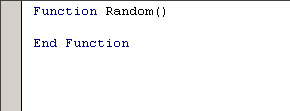4. Type the statement Random = Rnd as the body of the function.

The revised function should look like this:

`Function Random()   Random = Rnd  End Function `

The way you tell a function what value to return is by assigning a value to the name of the function, as if the function name were a variable. This function simply takes the value of the Visual Basic Rnd function and assigns it to the Random function.

5. Switch back to Excel, select cell A3, and then click the Insert Function button next to the formula bar.

Excel displays the Function Arguments window, which explains that the Random function doesn’t take any arguments.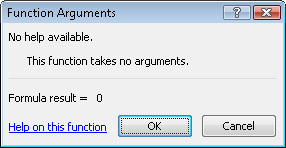6. Click OK to enter the random number into cell A3.

That’s all there is to creating a simple worksheet function. In the Visual Basic editor, you replace the word Sub with the word Function, and then somewhere in the function, you assign a value to the function name. In Excel, you put the function name into a formula, followed by parentheses.

### Add Arguments to a Custom Function

Suppose that in your worksheet, you want a formula that generates random whole numbers equal to 100 plus or minus 25. Or random whole numbers equal to 1000 plus or minus 100. The Excel RAND() function can’t give you that kind of random number. Neither, for that matter, can yours, but because yours is a custom function, you can add capabilities to it by adding arguments.

When you add arguments to a function, you need to decide what would make good arguments. Good arguments 1) provide just the right amount of information, 2) are general enough to allow you to do a lot of things with the function, and 3) don’t overlap in a confusing way. One good set of arguments for your Random function would consist of the following three arguments: one to specify the midpoint, one to specify the plus or minus range, and one to specify whether or not to round the final number. You can add those arguments to your function.

1. In the Visual Basic editor, type Midpoint, Range, Round between the parentheses after the name of the function.

The statement that contains the function name and its arguments is called the function declaration statement. In the function declaration statement, you declare the name of the function and also the names of all the arguments. The revised function declaration statement should look like this:

`Function Random(Midpoint, Range, Round) `

These three words are arguments to the function. You can use them inside the function as variables that have been prefilled with values.

2. Change the statement that assigns a value to the function name to this:

`Random = Rnd * (Range * 2) + (Midpoint – Range) `

To understand the formula, assume that the desired range is 25 and the desired midpoint is 100. That means you want random numbers that equal 100 plus or minus 25, or, in other words, random numbers between 75 and 125. The total spread is 50, which is two times the range. The Visual Basic Rnd function returns a random number between 0 and 1. Multiplying Rnd by Range * 2 gives you a random number between 0 and 50. To get numbers between 75 and 125, you need to add 75. Subtracting Range from Midpoint gives you the 75 that you need.

3. Insert these three statements to round the number if requested:

`If Round Then   Random = CLng(Random) End If `

In Visual Basic, a Long is an integer that can include large numbers. The Visual Basic function CLng converts a number to a Long, rounding it along the way. You round the random number only if the value of the Round argument is True. (Because the value of the Round argument already equals True or False, you don’t need to compare it to anything to get a conditional expression.) The complete function should look like this:

`Function Random(Midpoint, Range, Round)   Random = Rnd * (Range * 2) + (Midpoint – Range)   If Round Then   Random = CLng(Random)   End If End Function `
 Tip To see other functions that convert between data types, in the Visual Basic editor, click CLng, press F1, and look for Type Conversion Functions.
4. In Excel, enter 100 into cell B3, 25 into cell C3, and TRUE into cell D3.

You’ll use these values for the Midpoint, Range, and Round arguments of your function. The formula in cell C3 will turn into an error because it no longer matches the function declaration.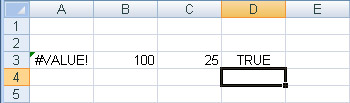5. Select cell A3, and click the Insert Function button next to the formula bar.

The Function Arguments window appears, showing you the three new arguments of your function.

6. Click in the Midpoint box, and click cell B3. Click in the Range box, and click cell C3. Click in the Round box, and click cell D3. Then click OK.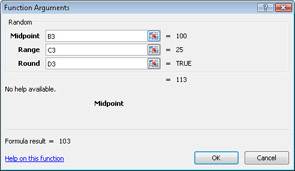After adjusting the formula, cell A3 contains a random number between 75 and 125. You use arguments to pass values to a function.

7. Change cell B3 to 1000 and cell C3 to 100.

The value of cell A3 changes to a random number between 900 and 1100. Whenever you change the value of a cell that the function refers to, the function calculates a new answer.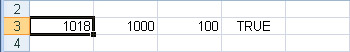Adding arguments is a way to make functions more flexible.

### Make a Function Volatile

Most functions recalculate only when the value of a cell that feeds into the function changes. Other functions (such as Excel’s RAND() function), are called volatile functions because they recalculate whenever any cell on the worksheet changes or whenever you press F9. You can make your function volatile so that it calculates a new random number whenever you press F9.

1. In Excel, press the F9 key repeatedly to see that the random number in cell A3 doesn’t change.

2. In the Visual Basic editor, insert this statement after the function declaration statement:

`Application.Volatile True`
3. Switch back to Excel, and press F9.

The random number in cell A3 changes. Press F9 several times to verify that the function generates random numbers in the range specified by the arguments.

Most of the time, you don’t want custom functions to be volatile. You want the function to recalculate only when a value that feeds into it changes. For those few cases in which you do want the formula to recalculate, just use the Application object’s Volatile method with True as an argument.

### Make Arguments Optional

The only problem with your new enhanced Random function is that it’s now more complicated to use in those simple cases in which you don’t need the new arguments. If you type =Random() in a cell without the arguments, Excel displays the #VALUE! error value. To avoid this error, you can tell Visual Basic that you want the arguments to be optional. Then you specify default values to use if an argument isn’t supplied.

1. In the Visual Basic editor, type the word Optional in front of each of the three argument names. (If you want, you can insert a line continuation character to split the statement onto two lines.)

The revised statement should look like this:

`Function Random(Optional Midpoint, _   Optional Range, Optional Round) `

You don’t have to make all the arguments optional, but once you make one argument optional, all the arguments that follow it must be optional as well. In other words, you place optional arguments at the end of the argument list.

1. In the Visual Basic editor, type = 0.5 after the word Midpoint, = 0.5 after the word Range, and = False after the word Round. Break the statement into two lines after the first comma.

The resulting statement should look like this:

`Function Random(Optional Midpoint = 0.5, _   Optional Range = 0.5, Optional Round = False) `

You can specify a default value for any optional argument. You assign the default value to the argument name in the same way you would assign a value to a variable-by using a simple equal sign.

1. In Excel, enter =Random() into cell A4.

A random number between 0 and 1 appears.

2. Delete any formulas in the worksheet that include the Random function (cells A3 and A4) so that you can step through other macros later in the chapter without stepping through the Random function.

Optional arguments allow you to add powerful features to a function while keeping it easy to use in cases in which you don’t need the extra features. To make an argument optional, add the word Optional before the argument name. To add a default value for an optional argument, assign the value to the argument name the same way you would if it were a variable.

### Use a Custom Function from a Macro

You can use a custom function from a macro just as easily as you can use it from a work-sheet cell.

1. In the Visual Basic editor, type Sub TestRandom at the bottom of the module, and then press Enter to start creating a macro.

2. Type MsgBox and a space.

Visual Basic displays the Quick Info box with the arguments for MsgBox.

3. Press Ctrl+Spacebar to show the list of global methods and properties, and then press the R key to scroll down to the words that begin with the letter R.Your Random function is automatically included in the list. Your function has the icon for a method next to it. Excel methods are simply functions built into Excel. You create new global methods by writing new functions.

4. Press the Tab key to insert the function name into the statement, and then type an opening parenthesis to begin the argument list.

Visual Basic displays the Quick Info box with the arguments for your custom function. The Quick Info box even shows the default values for the optional arguments.5. Type 200, 5, True as the list of arguments, and then type a closing parenthesis.

6. Press F5 to run the macro, and click OK when your random number appears.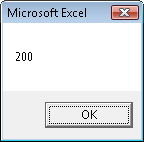A function is a procedure like a Sub procedure, except that it returns a value that you can use either in a cell in Excel or from a macro.

 Important A function used in a worksheet cell can include only those actions that can be executed while Excel is recalculating a worksheet. (Remember that some cells might even recalculate more than once.) Actions such as opening files or displaying message boxes can be included in functions that are called from macros, but if you include them in a function that’s called from a worksheet, the function simply returns the #VALUE! error value.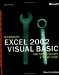Microsoft Excel 2002 Visual Basic for Applications Step by Step (Step by Step (Microsoft))
ISBN: 0735613591
EAN: 2147483647
Year: 2004
Pages: 99
Authors: Reed Jacobsen

Similar book on Amazon